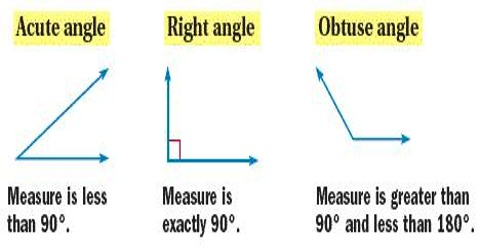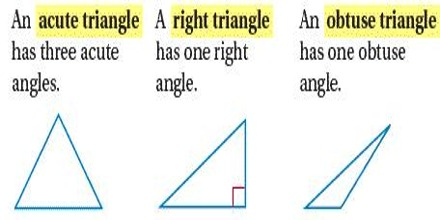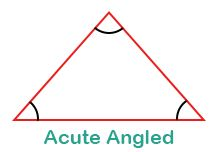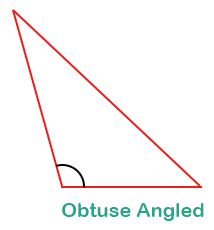Mathematic

# How to Identify Types of Triangles by Angles?Identifying Types of Triangles by Angles

The triangle is perhaps most common shape ever studied in geometry. It is a kind of polygon which has three sides. A triangle eventually has  three vertices as well. Types of triangles based on their angles

1. A RIGHT triangle has one 900
2. An OBTUSE triangle has one angle that is greater than 900.
3. An ACUTE triangle has all three angles less than 900.Right Triangles: A right triangle has one 90° and a variety of often-studied properties. When an angle of a triangle is right angle, i.e. 90°, it is termed as a right triangle or right-angled triangle. Its diagram in demonstrated below.Acute Triangle: The Acute Triangle has three acute angles (an acute angle measures less than 90°). A triangle is said to be an acute triangle or acute-angled triangle if all three angles are acute, i.e. less than 90°. The diagram of acute triangle is shown below.Obtuse Triangle: The Obtuse Triangle has an obtuse angle (an obtuse angle has more than 90°). In the picture on the left, the shaded angle is the obtuse angle that distinguishes this triangle Since the total degrees in any triangle are 180°, an obtuse triangle can only have one angle that measures more than 90°. If a triangle has one angle obtuse, i.e. greater than 90°, then it is called an obtuse triangle or obtuse-angled triangle which is shown in the following figure.